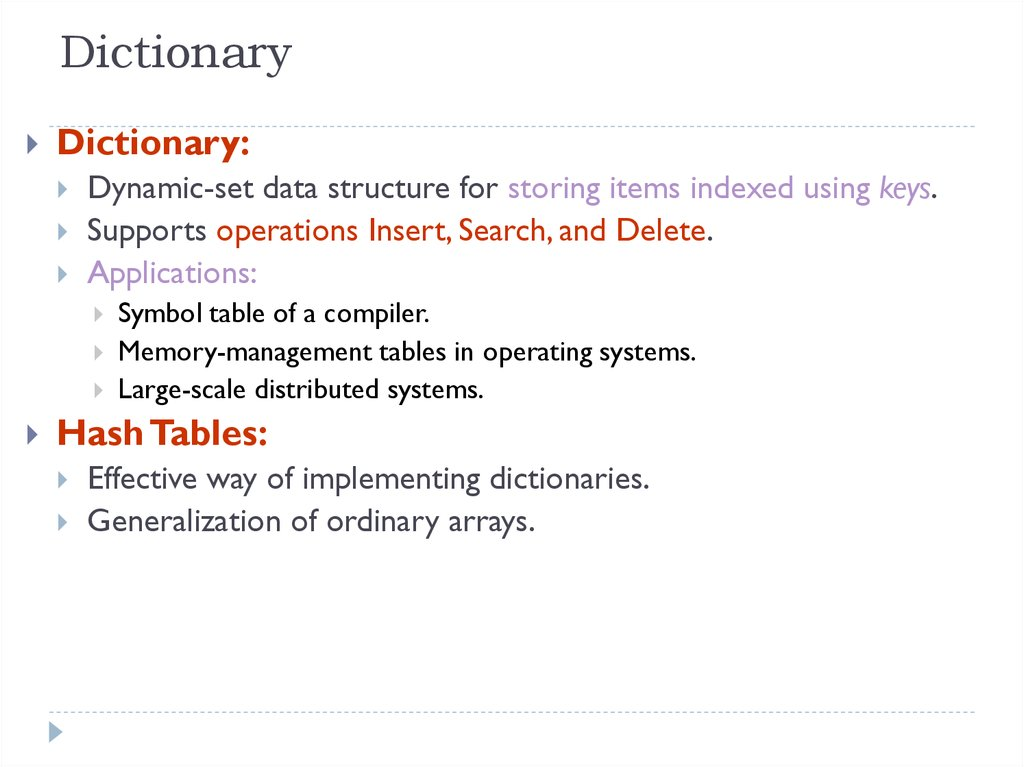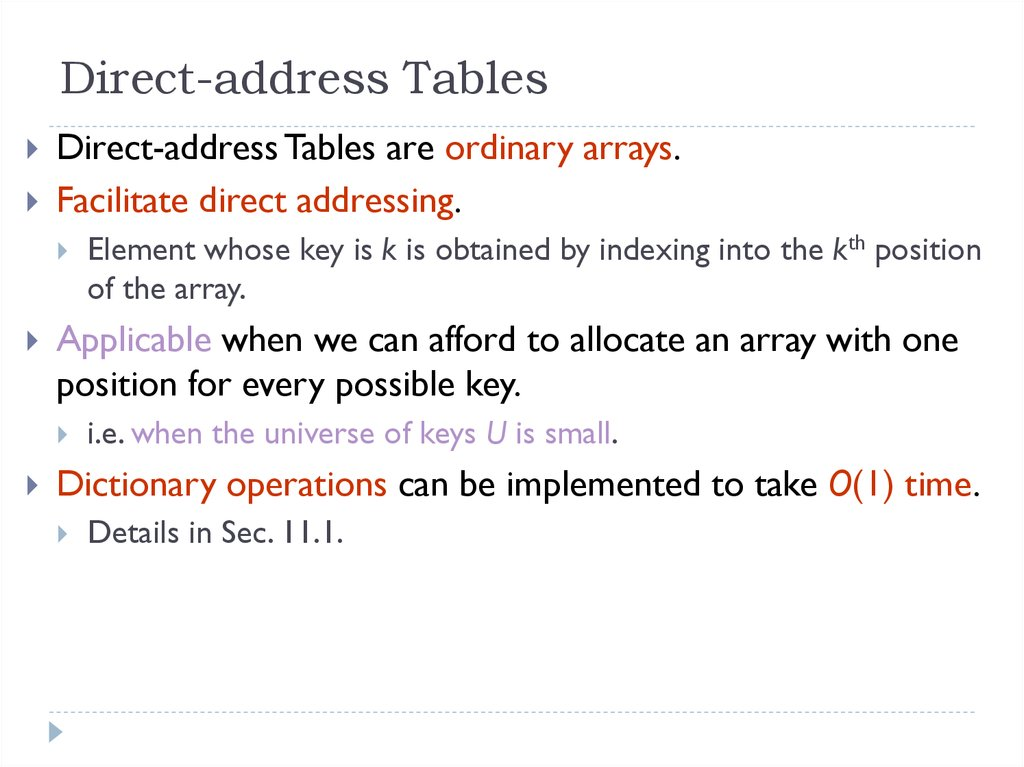# Hash Tables

SDP-4

## 2. Dictionary

Dictionary:
Dynamic-set data structure for storing items indexed using keys.
Supports operations Insert, Search, and Delete.
Applications:
Symbol table of a compiler.
Memory-management tables in operating systems.
Large-scale distributed systems.
Hash Tables:
Effective way of implementing dictionaries.
Generalization of ordinary arrays.

Applicable when we can afford to allocate an array with one
position for every possible key.
Element whose key is k is obtained by indexing into the kth position
of the array.
i.e. when the universe of keys U is small.
Dictionary operations can be implemented to take O(1) time.
Details in Sec. 11.1.

## 4. Hash Tables

Notation:
When U is very large,
U – Universe of all possible keys.
K – Set of keys actually stored in the dictionary.
|K| = n.
Arrays are not practical.
|K| << |U|.
Use a table of size proportional to |K| – The hash tables.
However, we lose the direct-addressing ability.
Define functions that map keys to slots of the hash table.

## 5. Hashing

Hash function h: Mapping from U to the slots of a hash table
T[0..m–1].
h : U {0,1,…, m–1}
With arrays, key k maps to slot A[k].
With hash tables, key k maps or “hashes” to slot T[h[k]].
h[k] is the hash value of key k.

## 6. Hashing

0
U
(universe of keys)
h(k1)
h(k4)
k1
K
(actual k2
keys)
k4
collision
k5
h(k2)=h(k5)
k3
h(k3)
m–1

## 7. Issues with Hashing

Multiple keys can hash to the same slot – collisions are
possible.
Design hash functions such that collisions are minimized.
But avoiding collisions is impossible.
Design collision-resolution techniques.
Search will cost Ө(n) time in the worst case.
However, all operations can be made to have an expected
complexity of Ө(1).

## 8. Methods of Resolution

Chaining:
Store all elements that hash to the same slot in a
the hash table slot.
All elements stored in hash table itself.
When collisions occur, use a systematic
(consistent) procedure to store elements in free
slots of the table.
0
k1
k4
k5
k2
k7
k3
k8
m–1
k6

## 9. Collision Resolution by Chaining

0
U
(universe of keys)
X
h(k1)=h(k4)
k1
k4
K
(actual
keys)
k2
k6
k5
X
h(k2)=h(k5)=h(k6)
k7
k8
k3
X
h(k3)=h(k7)
h(k8)
m–1

## 10. Collision Resolution by Chaining

0
U
(universe of keys)
k1
k4
k5
k2
k7
k3
k1
k4
K
(actual
keys)
k2
k6
k5
k7
k8
k3
k8
m–1
k6

## 11. Hashing with Chaining

Dictionary Operations:
Chained-Hash-Insert (T, x)
Chained-Hash-Delete (T, x)
Insert x at the head of list T[h(key[x])].
Worst-case complexity – O(1).
Delete x from the list T[h(key[x])].
Worst-case complexity – proportional to length of list with
Chained-Hash-Search (T, k)
Search an element with key k in list T[h(k)].
Worst-case complexity – proportional to length of list.

## 12. Analysis on Chained-Hash-Search

Load factor =n/m = average keys per slot.
m – number of slots.
n – number of elements stored in the hash table.
Worst-case complexity: (n) + time to compute h(k).
Average depends on how h distributes keys among m slots.
Assume
Simple uniform hashing.
Any key is equally likely to hash into any of the m slots, independent of
where any other key hashes to.
O(1) time to compute h(k).
Time to search for an element with key k is (|T[h(k)]|).
Expected length of a linked list = load factor = = n/m.

## 13. Expected Cost of an Unsuccessful Search

Theorem:
An unsuccessful search takes expected time Θ(1+α).
Proof:
Any key not already in the table is equally likely to hash to
any of the m slots.
To search unsuccessfully for any key k, need to search to
the end of the list T[h(k)], whose expected length is α.
Adding the time to compute the hash function, the total
time required is Θ(1+α).

## 14. Expected Cost of a Successful Search

Theorem:
A successful search takes expected time Θ(1+α).
Proof:
The probability that a list is searched is proportional to the number of
elements it contains.
Assume that the element being searched for is equally likely to be any of
the n elements in the table.
The number of elements examined during a successful search for an
element x is 1 more than the number of elements that appear before x in
x’s list.
These are the elements inserted after x was inserted.
Goal:
Find the average, over the n elements x in the table, of how many elements were
inserted into x’s list after x was inserted.

## 15. Expected Cost of a Successful Search

Theorem:
A successful search takes expected time Θ(1+α).
Proof (contd):
Let xi be the ith element inserted into the table, and let ki = key[xi].
Define indicator random variables Xij = I{h(ki) = h(kj)}, for all i, j.
Simple uniform hashing Pr{h(ki) = h(kj)} = 1/m
E[Xij] = 1/m.
Expected number of elements examined in a successful search is:
n
1 n
E 1 X ij
n i 1 j i 1
No. of elements inserted after xi into the same slot as xi.

## 16. Proof – Contd.

1 n
E 1
n i 1
X
ij
j i 1
1 n
1
n i 1
n
E
[
X
]
ij
j i 1
1 n
1
n i 1
1
j i 1m
n
(linearity of expectation)
n
1 n
1
(n i )
nm i 1
n
1 n
1
n i
nm i 1
i 1
1 2 n(n 1)
n
nm
2
n 1
1
2m
1
1
2
2n
Expected total time for a successful search
= Time to compute hash function + Time
to search
= O(2+ /2 – /2n) = O(1+ ).

## 17. Expected Cost – Interpretation

If n = O(m), then =n/m = O(m)/m = O(1).
Searching takes constant time on average.
Insertion is O(1) in the worst case.
Deletion takes O(1) worst-case time when lists are
Hence, all dictionary operations take O(1) time on
average with hash tables with chaining.

## 18. Good Hash Functions

Satisfy the assumption of simple uniform hashing.
Not possible to satisfy the assumption in practice.
Often use heuristics, based on the domain of the keys, to
create a hash function that performs well.
Regularity in key distribution should not affect uniformity.
Hash value should be independent of any patterns that might
exist in the data.
E.g. Each key is drawn independently from U according to a
probability distribution P:
k:h(k) = j P(k) = 1/m
for j = 0, 1, … , m–1.
An example is the division method.

## 19. Keys as Natural Numbers

Hash functions assume that the keys are natural numbers.
When they are not, have to interpret them as natural
numbers.
Example: Interpret a character string as an integer expressed
in some radix notation. Suppose the string is CLRS:
ASCII values: C=67, L=76, R=82, S=83.
There are 128 basic ASCII values.
So, CLRS = 67·1283+76 ·1282+ 82·1281+ 83·1280
= 141,764,947.

## 20. Division Method

Map a key k into one of the m slots by taking the
remainder of k divided by m. That is,
h(k) = k mod m
Example: m = 31 and k = 78 h(k) = 16.
Advantage: Fast, since requires just one division
operation.
Disadvantage: Have to avoid certain values of m.
Don’t pick certain values, such as m=2p
Or hash won’t depend on all bits of k.
Good choice for m:
Primes, not too close to power of 2 (or 10) are good.

## 21. Multiplication Method

If 0 < A < 1, h(k) = m (kA mod 1) = m (kA – kA )
where kA mod 1 means the fractional part of kA, i.e., kA –
kA .
Disadvantage: Slower than the division method.
Advantage:Value of m is not critical.
Typically chosen as a power of 2, i.e., m = 2p, which makes
implementation easy.
Example: m = 1000, k = 123, A 0.6180339887…
h(k) = 1000(123 · 0.6180339887 mod 1)
= 1000 · 0.018169... = 18.

## 22. Multiplication Mthd. – Implementation

Choose m = 2p, for some integer p.
Let the word size of the machine be w bits.
Assume that k fits into a single word. (k takes w bits.)
Let 0 < s < 2w. (s takes w bits.)
Restrict A to be of the form s/2w.
Let k s = r1 ·2w+ r0 .
r1 holds the integer part of kA ( kA ) and r0 holds the fractional part
of kA (kA mod 1 = kA – kA ).
We don’t care about the integer part of kA.
So, just use r0, and forget about r1.

## 23. Multiplication Mthd – Implementation

w bits
k
binary point
r1
s = A·2w
·
r0
extract p bits
h(k)
We want m (kA mod 1) . We could get that by shifting r0 to the
left by p = lg m bits and then taking the p bits that were shifted to
the left of the binary point.
But, we don’t need to shift. Just take the p most significant bits of
r0.

## 24. How to choose A?

Another example: On board.
How to choose A?
The multiplication method works with any legal value of A.
But it works better with some values than with others, depending
on the keys being hashed.
Knuth suggests using A ( 5 – 1)/2.